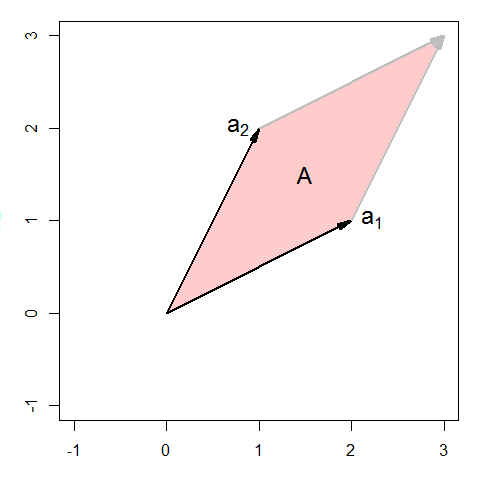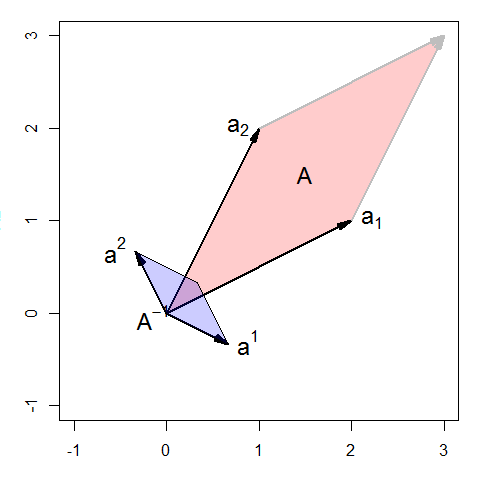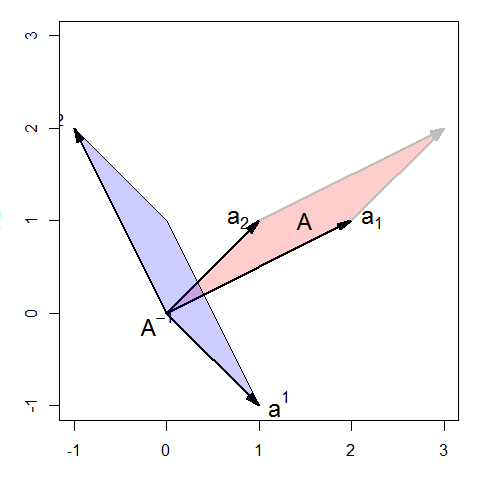# Inverse of a matrix

#### May 29, 2019

The inverse of a matrix plays the same roles in matrix algebra as the reciprocal of a number and division does in ordinary arithmetic: Just as we can solve a simple equation like $$4 x = 8$$ for $$x$$ by multiplying both sides by the reciprocal $4 x = 8 \Rightarrow 4^{-1} 4 x = 4^{-1} 8 \Rightarrow x = 8 / 4 = 2$ we can solve a matrix equation like $$\mathbf{A x} = \mathbf{b}$$ for the vector $$\mathbf{x}$$ by multiplying both sides by the inverse of the matrix $$\mathbf{A}$$, $\mathbf{A x} = \mathbf{b} \Rightarrow \mathbf{A}^{-1} \mathbf{A x} = \mathbf{A}^{-1} \mathbf{b} \Rightarrow \mathbf{x} = \mathbf{A}^{-1} \mathbf{b}$

The following examples illustrate the basic properties of the inverse of a matrix.

### Load the matlib package

This defines: inv(), Inverse(); the standard R function for matrix inverse is solve()

library(matlib)

### Create a 3 x 3 matrix

The ordinary inverse is defined only for square matrices.

    A <- matrix( c(5, 1, 0,
3,-1, 2,
4, 0,-1), nrow=3, byrow=TRUE)
det(A)
##  16

## Basic properties

### 1. det(A) != 0, so inverse exists

Only non-singular matrices have an inverse.

   (AI  <- inv(A))
##        [,1]    [,2]   [,3]
## [1,] 0.0625  0.0625  0.125
## [2,] 0.6875 -0.3125 -0.625
## [3,] 0.2500  0.2500 -0.500

### 2. Definition of the inverse: $$A^{-1} A = A A^{-1} = I$$ or AI * A = diag(nrow(A))

The inverse of a matrix $$A$$ is defined as the matrix $$A^{-1}$$ which multiplies $$A$$ to give the identity matrix, just as, for a scalar $$a$$, $$a a^{-1} = a / a = 1$$.

NB: Sometimes you will get very tiny off-diagonal values (like 1.341e-13). The function zapsmall() will round those to 0.

   AI %*% A
##      [,1] [,2] [,3]
## [1,]    1    0    0
## [2,]    0    1    0
## [3,]    0    0    1

### 3. Inverse is reflexive: inv(inv(A)) = A

Taking the inverse twice gets you back to where you started.

   inv(AI)
##      [,1] [,2] [,3]
## [1,]    5    1    0
## [2,]    3   -1    2
## [3,]    4    0   -1

### 4. inv(A) is symmetric if and only if A is symmetric

   inv( t(A) )
##        [,1]    [,2]  [,3]
## [1,] 0.0625  0.6875  0.25
## [2,] 0.0625 -0.3125  0.25
## [3,] 0.1250 -0.6250 -0.50
   is_symmetric_matrix(A)
##  FALSE
   is_symmetric_matrix( inv( t(A) ) )
##  FALSE

Here is a symmetric case:

   B <- matrix( c(4, 2, 2,
2, 3, 1,
2, 1, 3), nrow=3, byrow=TRUE)
inv(B)
##       [,1]  [,2]  [,3]
## [1,]  0.50 -0.25 -0.25
## [2,] -0.25  0.50  0.00
## [3,] -0.25  0.00  0.50
   inv( t(B) )
##       [,1]  [,2]  [,3]
## [1,]  0.50 -0.25 -0.25
## [2,] -0.25  0.50  0.00
## [3,] -0.25  0.00  0.50
   is_symmetric_matrix(B)
##  TRUE
   is_symmetric_matrix( inv( t(B) ) )
##  TRUE
   all.equal( inv(B), inv( t(B) ) )
##  TRUE

## More properties of matrix inverse

### 1. inverse of diagonal matrix = diag( 1/ diagonal)

In these simple examples, it is often useful to show the results of matrix calculations as fractions, using MASS::fractions().

   D <- diag(c(1, 2, 4))
inv(D)
##      [,1] [,2] [,3]
## [1,]    1  0.0 0.00
## [2,]    0  0.5 0.00
## [3,]    0  0.0 0.25
   MASS::fractions( diag(1 / c(1, 2, 4)) )
##      [,1] [,2] [,3]
## [1,]   1    0    0
## [2,]   0  1/2    0
## [3,]   0    0  1/4

### 2. Inverse of an inverse: inv(inv(A)) = A

   A <- matrix(c(1, 2, 3,  2, 3, 0,  0, 1, 2), nrow=3, byrow=TRUE)
AI <- inv(A)
inv(AI)
##      [,1] [,2] [,3]
## [1,]    1    2    3
## [2,]    2    3    0
## [3,]    0    1    2

### 3. inverse of a transpose: inv(t(A)) = t(inv(A))

   inv( t(A) )
##       [,1] [,2]  [,3]
## [1,]  1.50 -1.0  0.50
## [2,] -0.25  0.5 -0.25
## [3,] -2.25  1.5 -0.25
   t( inv(A) )
##       [,1] [,2]  [,3]
## [1,]  1.50 -1.0  0.50
## [2,] -0.25  0.5 -0.25
## [3,] -2.25  1.5 -0.25

### 4. inverse of a scalar * matrix: inv( k*A ) = (1/k) * inv(A)

   inv(5 * A)
##      [,1]  [,2]  [,3]
## [1,]  0.3 -0.05 -0.45
## [2,] -0.2  0.10  0.30
## [3,]  0.1 -0.05 -0.05
   (1/5) * inv(A)
##      [,1]  [,2]  [,3]
## [1,]  0.3 -0.05 -0.45
## [2,] -0.2  0.10  0.30
## [3,]  0.1 -0.05 -0.05

### 5. inverse of a matrix product: inv(A * B) = inv(B) %*% inv(A)

   B <- matrix(c(1, 2, 3, 1, 3, 2, 2, 4, 1), nrow=3, byrow=TRUE)
C <- B[, 3:1]
A %*%  B
##      [,1] [,2] [,3]
## [1,]    9   20   10
## [2,]    5   13   12
## [3,]    5   11    4
   inv(A %*%  B)
##      [,1]  [,2]  [,3]
## [1,]  4.0 -1.50 -5.50
## [2,] -2.0  0.70  2.90
## [3,]  0.5 -0.05 -0.85
   inv(B) %*%  inv(A)
##      [,1]  [,2]  [,3]
## [1,]  4.0 -1.50 -5.50
## [2,] -2.0  0.70  2.90
## [3,]  0.5 -0.05 -0.85

This extends to any number of terms: the inverse of a product is the product of the inverses in reverse order.

   (ABC <- A %*% B %*% C)
##      [,1] [,2] [,3]
## [1,]   77  118   49
## [2,]   53   97   42
## [3,]   41   59   24
   inv(A %*% B %*% C)
##      [,1]  [,2]   [,3]
## [1,]  1.5 -0.59  -2.03
## [2,] -4.5  1.61   6.37
## [3,]  8.5 -2.95 -12.15
   inv(C) %*% inv(B) %*% inv(A)
##      [,1]  [,2]   [,3]
## [1,]  1.5 -0.59  -2.03
## [2,] -4.5  1.61   6.37
## [3,]  8.5 -2.95 -12.15
   inv(ABC)
##      [,1]  [,2]   [,3]
## [1,]  1.5 -0.59  -2.03
## [2,] -4.5  1.61   6.37
## [3,]  8.5 -2.95 -12.15

### 6. $$\det (A^{-1}) = 1 / \det(A) = [\det(A)]^{-1}$$

The determinant of an inverse is the inverse (reciprocal) of the determinant

  det(AI)
##  0.25
  1 / det(A)
##  0.25

## Geometric interpretations

Some of these properties of the matrix inverse can be more easily understood from geometric diagrams. Here, we take a $$2 \times 2$$ non-singular matrix $$A$$,

A <- matrix(c(2, 1,
1, 2), nrow=2, byrow=TRUE)
A
##      [,1] [,2]
## [1,]    2    1
## [2,]    1    2
det(A)
##  3

The larger the determinant of $$A$$, the smaller is the determinant of $$A^{-1}$$.

AI <- inv(A)
MASS::fractions(AI)
##      [,1] [,2]
## [1,]  2/3 -1/3
## [2,] -1/3  2/3
det(AI)
##  0.3333

Now, plot the rows of $$A$$ as vectors $$a_1, a_2$$ from the origin in a 2D space. As illustrated in vignette("det-ex1"), the area of the parallelogram defined by these vectors is the determinant.

par(mar=c(3,3,1,1)+.1)
xlim <- c(-1,3)
ylim <- c(-1,3)
plot(xlim, ylim, type="n", xlab="X1", ylab="X2", asp=1)
sum <- A[1,] + A[2,]
# draw the parallelogram determined by the rows of A
polygon( rbind(c(0,0), A[1,], sum, A[2,]), col=rgb(1,0,0,.2))
vectors(A, labels=c(expression(a), expression(a)), pos.lab=c(4,2))
vectors(sum, origin=A[1,], col="gray")
vectors(sum, origin=A[2,], col="gray")
text(mean(A[,1]), mean(A[,2]), "A", cex=1.5)The rows of the inverse $$A^{-1}$$ can be shown as vectors $$a^1, a^2$$ from the origin in the same space.

vectors(AI, labels=c(expression(a^1), expression(a^2)), pos.lab=c(4,2))
sum <- AI[1,] + AI[2,]
polygon( rbind(c(0,0), AI[1,], sum, AI[2,]), col=rgb(0,0,1,.2))
text(mean(AI[,1])-.3, mean(AI[,2])-.2, expression(A^{-1}), cex=1.5)Thus, we can see:

• The shape of $$A^{-1}$$ is a $$90^o$$ rotation of the shape of $$A$$.

• $$A^{-1}$$ is small in the directions where $$A$$ is large.

• The vector $$a^2$$ is at right angles to $$a_1$$ and $$a^1$$ is at right angles to $$a_2$$

• If we multiplied $$A$$ by a constant $$k$$ to make its determinant larger (by a factor of $$k^2$$), the inverse would have to be divided by the same factor to preserve $$A A^{-1} = I$$.

One might wonder whether these properties depend on symmetry of $$A$$, so here is another example, for the matrix A <- matrix(c(2, 1, 1, 1), nrow=2), where $$\det(A)=1$$.

(A <- matrix(c(2, 1, 1, 1), nrow=2))
##      [,1] [,2]
## [1,]    2    1
## [2,]    1    1
(AI <- inv(A))
##      [,1] [,2]
## [1,]    1   -1
## [2,]   -1    2

The areas of the two parallelograms are the same because $$\det(A) = \det(A^{-1}) = 1$$.